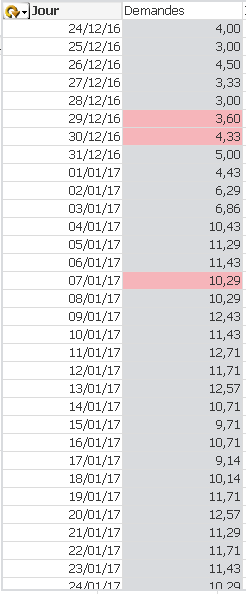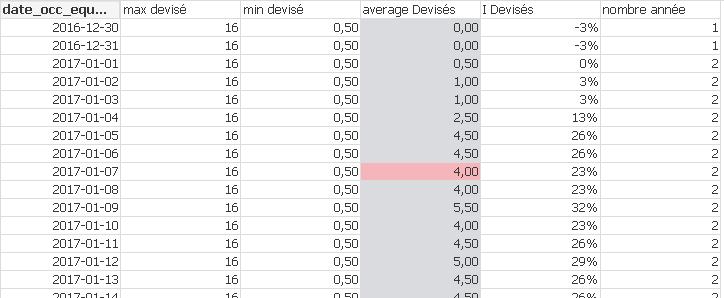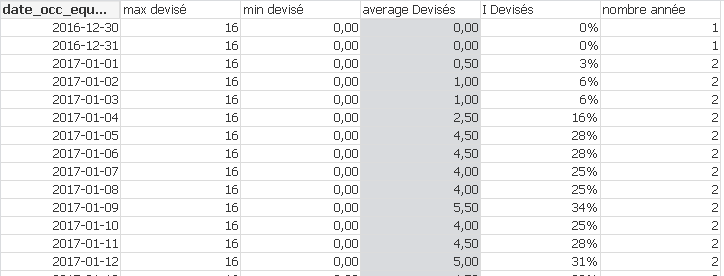# QlikView App Dev

Discussion Board for collaboration related to QlikView App Development.

Announcements
cancel
Showing results for
Did you mean:Contributor II

## Min or Max of a calculated expression

Hello,

I have been struggling too long so I ask for your kind help.

My goal is to fill in a variable with the max of the following expression. I need to get a single value at the end.

I have a table with 2 columns

dimension: it'a cyclical group with the first dimension equal to Jour=Date(date_occ-(year(date_occ)-v_AnneeRef)*364,'DD/MM/YY')

It is used to compare equivalent day between different years. A monday with a monday A tuesday with a tuesday etc

So I have several date_occ already aggregated under each "jour"

Expressions:

Demande: =(\$(vExpr_nombrededemandes))/count(DISTINCT{\$< statut_option= , statut_affaire={"*"}> } ANNEE_OCCUPATION)

vExpr_nombrededemandes= (Count({\$< libel_segment={"*"}-{'Salles expos/orgas'}-{'Autres'}, statut_option= , statut_affaire={"*"}-{'IMMOBIL'}>}  DISTINCT mot_directeur) )Thanks a lot

26 RepliesContributor II
Author

I identified the issue. but don't know how to solve.

My problem is that when I filter  on "statut_affaire", my MIN goes from 0 to 0,5 even if the min average should be 0 as it appears on the 2016-12-30.

Min= (Min(TOTAL Aggr((\$(vExpr_Nombrededevisés)/(count(DISTINCT{\$< statut_option= , statut_affaire={"*"}> } ANNEE_OCCUPATION))), [date_occ_equiv_2017])))

vExpr_Nombrededevisés= (count(  {\$< statut_affaire={"*"}-{'IMMOBIL'}, statut_option= ,libel_segment={"*"}-{'Salles expos/orgas'}-{'Autres'}, numero_dernier_devis={'*'}-{'0'} >}  DISTINCT mot_directeur) )

average devisés= \$(vExpr_Nombrededevisés)/\$(VExpr_nombreannée)

VExpr_nombreannée= (count(DISTINCT {\$< statut_option= , statut_affaire={"*"}> } ANNEE_OCCUPATION ))

with a filter on statut_affairewithout a filterthanksMVP

(Min({\$< statut_option= , statut_affaire={"*"}>} TOTAL Aggr((\$(vExpr_Nombrededevisés)/(count(DISTINCT{\$< statut_option= , statut_affaire={"*"}> } ANNEE_OCCUPATION))), [date_occ_equiv_2017])))Contributor II
Author

OK

You are the boss!!

As far as now, everything is okay it answers my need!

I might need some help later, but it will be on an other topic!!

THANKSMVP

Super awesome!!

Best,

SunnyMVP

Why 'Assumed Answer' my friend? I thought we do have a correct and so many helpful responses on this post... isn't it?Contributor II
Author

Because I only found this possibility. How can I close?MVP